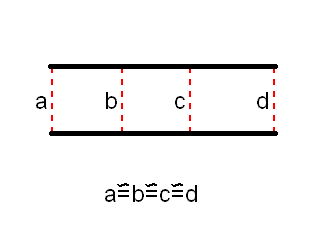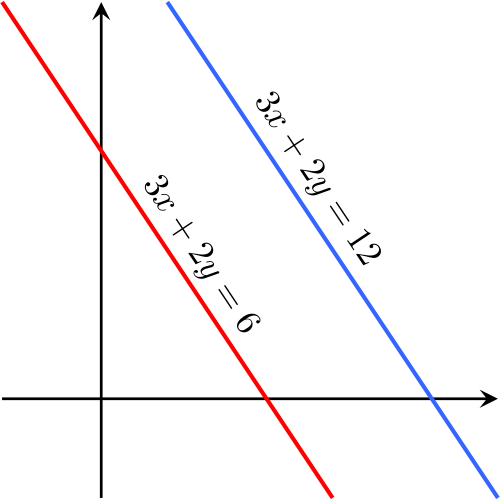# parallel line

## What Are Parallel Lines?

Parallel lines are two lines that are always the same distance apart and never touch. In order for two lines to be parallel, they must be drawn in the same plane, a perfectly flat surface like a wall or sheet of paper.

Parallel lines are useful in understanding the relationships between paths of objects and sides of various shapes. For example, squares, rectangles, and parallelograms have sides across from each other that are parallel.

In formulas, parallel lines are indicated with a pair of vertical pipes between the line names, like this:

AB || CD

Each line has many parallels. Any line that has the same slope as the original will never intersect with it. Lines that would never cross, even if extended forever, are parallel.

## Railroad Tracks and Parallel Lines

Sometimes it is helpful to think of parallel lines as a set of railroad tracks. The two rails of the track are created for the wheels on each side of the train to travel along. Because the wheels of the train are always the same distance apart, they do not get closer, not even when they turn.The tracks have to be the same distance apart everywhere. Since they are made by humans, railroad tracks aren’t quite parallel, but in order to work properly, they have to be awfully close.

The other difference between railroad tracks and perfectly parallel lines is that tracks are built over hills and valleys. The ground they cover is not perfectly flat. When mathematicians imagine parallel lines, on the other hand, they draw them on a perfectly flat surface.

## Graphing Parallel Lines

When two parallel lines are graphed, they must always be at the same angle, which means they’ll always have the same slope, or steepness.

Here’s a graph of two parallel lines:Even though these two lines don’t start in the same place, you can see that they are equally steep. They decrease or slope downward at the same rate. That ensures that they’re parallel.

If you look at the equations of those two lines, you may notice something: they are exactly the same except for the numbers on the right hand side, the ‘6’ and ’12.’ We can use some simple algebra and rewrite the two equations in slope-intercept form (y = mx + b), a form of a line that is the most familiar to people.

The blue line’s equation will then be:

= -3/2+ 6

While the red line will have an equation of:

y = -3/2x + 3

Again, you might notice that the two equations are exactly the same except for one thing: the number to the right of x. That number is called the constant and it tells us how high or low the line sits on the graph. When the line is in slope-intercept form, that number also tells us the y-intercept which is exactly where the line hits the y-axis, the vertical line marking zero on the graph. In this graph, the two lines are exactly the same except that one of them

## One thought on “parallel line”

1.19703 says:

wow เยี่ยม